# Capacitor and Capacitance Simplified & Explained

## What is a Capacitor?

Capacitor is a device that stores electric charge. A capacitor consists of two conductors separated by an insulator. Capacitors have many applications some of them include Computer RAM memory and keyboards, Electronic flashes for cameras, Electric power surge protectors and Radios and electronic circuits.

Another definition of capacitor can be that it is an electronic component having two conducting surfaces separated by an insulating material that is used for storing the charge. Capacitor is used for time delays, tuned circuits and filters.

### Types of Capacitors

There are three types of capacitors:

• Parallel-Plate Capacitor
• Cylindrical Capacitor
• Spherical Capacitor

A cylindrical capacitor is a parallel-plate capacitor that has been rolled up with an insulating layer between the plates. A spherical capacitor is a kind of capacitor which have one or more thin hollow spherical plate conductors. Also types of capacitors depend upon the insulating material used air, ceramic, mica or glass etc.

Capacitors and Capacitance

Capacitance is the ability of a body to store an electrical charge. Any object that can be electrically charged exhibits capacitance. A common form of energy storage device is a parallel-plate capacitor.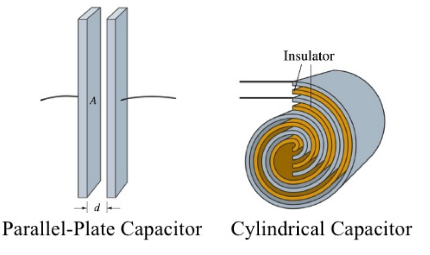A capacitor is a device for storing electrical charge. Capacitors consist of a pair of conducting plates separated by an insulating material (oil, paper, air). The measure of the extent to which a capacitor can store charge is called Capacitance. Capacitance is measured in farads F, or more usually microfarads mF or picofarads pF.

The stored charge Q of a capacitor is proportional to the potential difference V between the plates and the capacitance C of a capacitor is the constant of proportionality which is measured in Farads.

### Working of Capacitor

One plate is joined to the positive end of the supply and the other to the negative end or is earthed. When such a capacitor is put across a battery, there is a momentary flow of electrons from A to B. As negatively-charged electrons are withdrawn from A, it becomes positive and as these electrons collect on B, it becomes negative. Hence, a potential difference  is established between plates A and B.

The transient flow of electrons gives rise to charging current. The strength of the charging current is maximum when the two plates are uncharged but it then decreases and finally ceases when potential difference across the plates becomes slowly and slowly equal and opposite to the battery e.m.f.

#### Capacitors in Series

When we put capacitors in series the same charge appears on all capacitors and total Voltage is the sum of the individual voltages.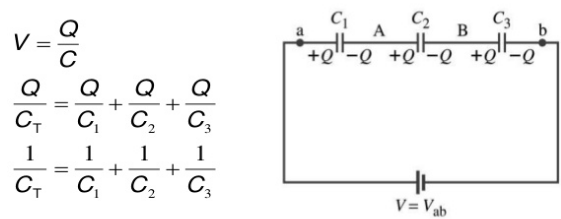#### Capacitors in Parallel

In the case of capacitors in parallel the total charge on the capacitors is the sum of all the charges. The total capacitance of capacitors in parallel is the sum of their individual capacitance’s.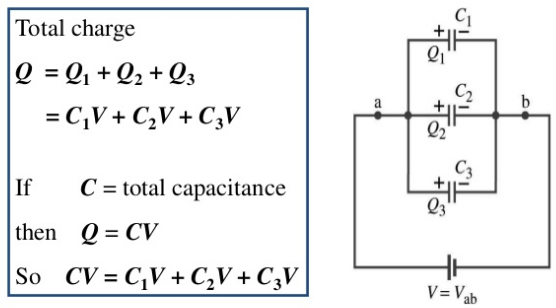#### Factors Affecting Capacitance

The capacitance is only a function of the physical dimensions (geometry) of the conductors and the permittivity of the dielectric material used in a capacitor. There are three basic factors of capacitor construction determining the amount of capacitance created i.e plate area, plates spacing and dielectric material used.

C = εr  ε0 A / d

C is the capacitance

A is the area of overlap of the two plates

εr is the relative permittivity of the material between plates

ε0 is the electric constant (ε0 ≈ 8.854×10−12 F m–1)

and d is the separation between the plates

A capacitor in the form of a simple electric circuit is shown below.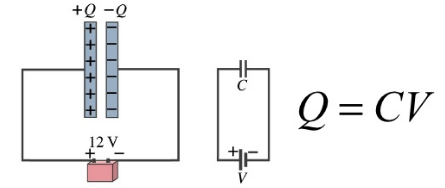Parallel-Plate Capacitor

A simple parallel-plate capacitor consists of two conducting plates of area A separated by a distance d . Charge +Q is placed on one plate and –Q on the other plate. An electric field E is created between the plates.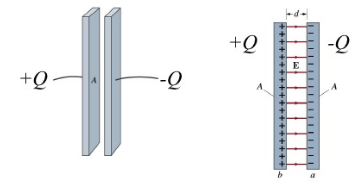While charging a capacitor the current flow is high initially but it reduces and reaches to zero finally when the capacitor is fully charged. Other factors that can affect the charging include capacitance and resistance. Similarly the rate of discharge is high because the potential difference across the plates is large. As the potential difference falls, so too does the current flowing.

When charging a capacitor, work has to be done against:

a. attractive forces when removing electrons from the positive plate.

b. repulsive forces when adding electrons to the negative plate.

The work is stored as potential energy in the electric field between the plates.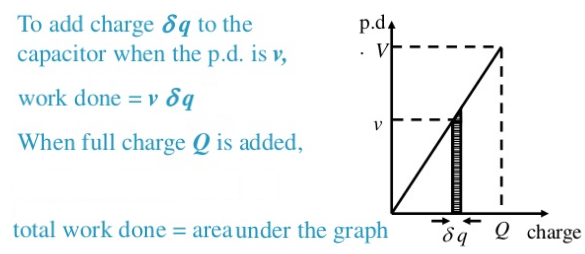#### Applications of Capacitors

A capacitor can store electric energy when disconnected from its charging circuit, so it can be used like a temporary battery. Capacitors are commonly used in electronic devices to maintain power supply while batteries are being changed.

DC blocking capacitor: In this application the capacitor blocks the passage of DC current (after completely charged) and yet allows the AC to pass at certain portion of a circuit.

Capacitor as a filter: Capacitors are the main elements of filters. There are several types of filters that are used in electronic circuits, such as Low Pass Filter, High Pass Filter, Band Pass Filter etc.

Capacitor as a discharge unit: Capacitors used as a charging unit and the release of the charge (discharge energy) is used for triggering, ignition, and in high scale as a power source.

By Pass capacitor: The reactance of capacitor decreases as the frequency increases. Therefore in certain application it is used in parallel with other components to bypass it at a specified frequency.

Coupling capacitor: The ability of capacitor to pass AC signal, allows it to couple a section of an electronic circuit to another circuit.

Decoupling capacitor: In high speed electronic logic switching causes draw of significant amount of current which in turn would cause disturbance in the logic voltage level. Decoupling capacitor is typically located very close to the IC output and serves as a local energy source to provide the needed extra current and therefore minimizes the noise and disturbances to the logic signal.